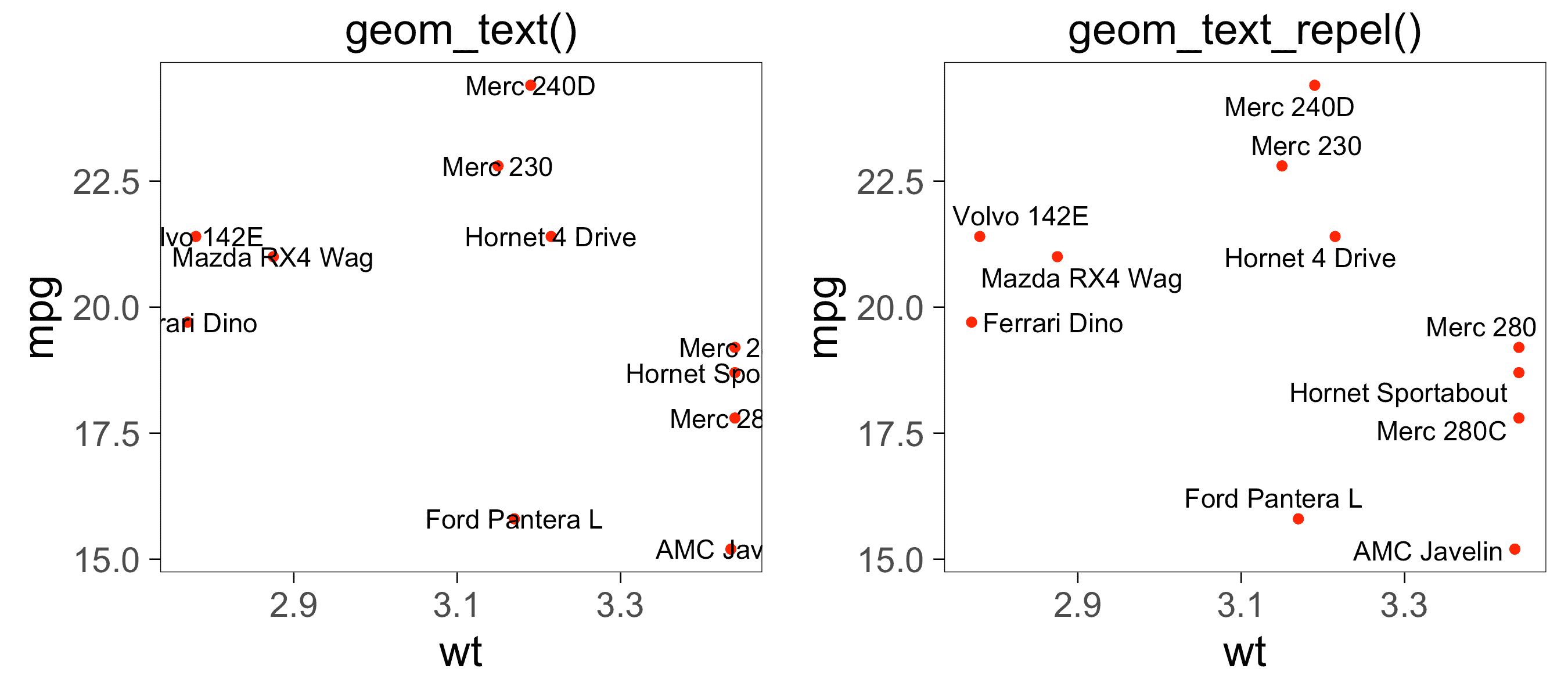# Getting started with ggrepel

## Overview

ggrepel provides geoms for ggplot2 to repel overlapping text labels:

• `geom_text_repel()`
• `geom_label_repel()`

Text labels repel away from each other, away from data points, and away from edges of the plotting area (panel).

Let’s compare `geom_text()` and `geom_text_repel()`:

``````library(ggrepel)
set.seed(42)

dat <- subset(mtcars, wt > 2.75 & wt < 3.45)
dat\$car <- rownames(dat)

p <- ggplot(dat, aes(wt, mpg, label = car)) +
geom_point(color = "red")

p1 <- p + geom_text() + labs(title = "geom_text()")

p2 <- p + geom_text_repel() + labs(title = "geom_text_repel()")

gridExtra::grid.arrange(p1, p2, ncol = 2)``````## Installation

ggrepel is available on CRAN:

``install.packages("ggrepel")``

The latest development version may have new features, and you can get it from GitHub:

``````# Use the devtools package
# install.packages("devtools")
devtools::install_github("slowkow/ggrepel")``````